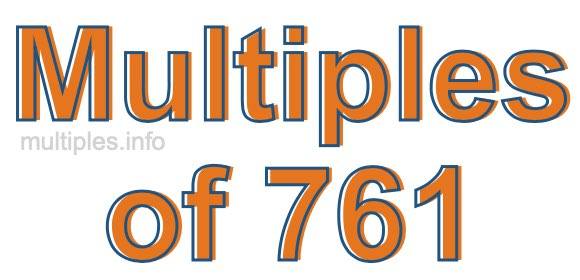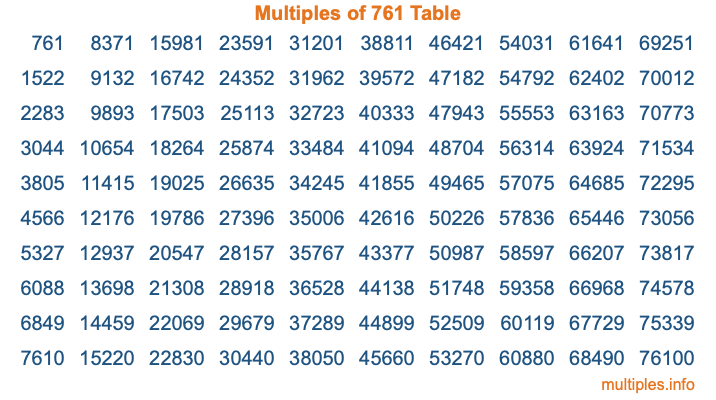Multiples of 761Welcome to the Multiples of 761 page. Here we will first teach you everything you will ever need to know about the multiples of 761, and then give you a study guide summary of everything we taught you to make sure you remember it all. Use this page to look up facts and learn information about the multiples of 761. This page will make you a multiples of seven hundred sixty-one expert!

Definition of Multiples of 761
Multiples of 761 are all the numbers that when divided by 761 equal an integer. Each of the multiples of 761 are called a multiple. A multiple of 761 is created by multiplying 761 by an integer.

Therefore, to create a list of multiples of 761, you start with 1 multiplied by 761, then 2 multiplied by 761, then 3 multiplied by 761, and so on for as long as you want. Thus, the list of the first five multiples of 761 is 761, 1522, 2283, 3044, and 3805. To see a larger list of multiples of 761, see the printable image of Multiples of 761 further down on this page. We also have a category where you can choose any nth multiple of 761.

Multiples of 761 Checker
The Multiples of 761 Checker below checks to see if any number of your choice is a multiple of 761. In other words, it checks to see if there is any number (integer) that when multiplied by 761 will equal your number. To do that, we divide your number by 761. If the the quotient is an integer, then your number is a multiple of 761.

Is  a multiple of 761?

Least Common Multiple of 761 and ...
A Least Common Multiple (LCM) is the lowest multiple that two or more numbers have in common. This is also called the smallest common multiple or lowest common multiple and is useful to know when you are adding our subtracting fractions. Enter one or more numbers below (761 is already entered) to find the LCM.

Check out our LCM Calculator if you need more details about the Least Common Multiple or if you need the LCM for different numbers for adding and subtraction fractions.

nth Multiple of 761
As we stated above, 761 is the first multiple of 761, 1522 is the second multiple of 761, 2283 is the third multiple of 761, and so on. Enter a number below to find the nth multiple of 761.

th multiple of 761

Multiples of 761 vs Factors of 761
761 is a multiple of 761 and a factor of 761, but that is where the similarities end. All postive multiples of 761 are 761 or greater than 761. All positive factors of 761 are 761 or less than 761.

Below is the beginning list of multiples of 761 and the factors of 761 so you can compare:

Multiples of 761: 761, 1522, 2283, 3044, 3805, etc.

Factors of 761: 1, 761

As you can see, the multiples of 761 are all the numbers that you can divide by 761 to get a whole number. The factors of 761, on the other hand, are all the whole numbers that you can multiply by another whole number to get 761.

It's also interesting to note that if a number (x) is a factor of 761, then 761 will also be a multiple of that number (x).

Multiples of 761 vs Divisors of 761
The divisors of 761 are all the integers that 761 can be divided by evenly. Below is a list of the divisors of 761.

Divisors of 761: 1, 761

The interesting thing to note here is that if you take any multiple of 761 and divide it by a divisor of 761, you will see that the quotient is an integer.

Multiples of 761 Table
Below is an image of the first 100 multiples of 761 in a table. The table is in chronological order, column by column. The first column has the first ten multiples of 761, the second column has the next ten multiples of 761, and so on.The Multiples of 761 Table is also referred to as the 761 Times Table or Times Table of 761. You are welcome to print out our table for your studies.

Negative Multiples of 761
Although not often discussed or needed in math, it is worth mentioning that you can make a list of negative multiples of 761 by multiplying 761 by -1, then by -2, then by -3, and so on, to get the following list of negative multiples of 761:

-761, -1522, -2283, -3044, -3805, etc.

Multiples of 761 Summary
Below is a summary of important Multiples of 761 facts that we have discussed on this page. To retain the knowledge on this page, we recommend that you read through the summary and explain to yourself or a study partner why they hold true.

There are an infinite number of multiples of 761.

A multiple of 761 divided by 761 will equal a whole number.

761 divided by a factor of 761 equals a divisor of 761.

The nth multiple of 761 is n times 761.

The largest factor of 761 is equal to the first positive multiple of 761.

761 is a multiple of every factor of 761.

761 is a multiple of 761.

A multiple of 761 divided by a divisor of 761 equals an integer.

761 divided by a divisor of 761 equals a factor of 761.

Any integer times 761 will equal a multiple of 761.

Multiples of a Number
Here you can get the multiples of another number, all with the same attention to detail as we did for multiples of 761 on this page.

Multiples of
Multiples of 762
Did you find our page about multiples of seven hundred sixty-one educational? Do you want more knowledge? Check out the multiples of the next number on our list!